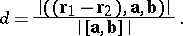# Skew lines

(diff) ← Older revision | Latest revision (diff) | Newer revision → (diff)

Two straight lines in space that do not lie in a plane. The angle between two skew lines is defined as either of the angles between any two lines parallel to them and passing through a point of space. Ifand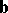are the direction vectors of two skew lines, then the cosine of the angle between them is given by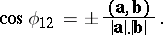The common perpendicular of two skew lines is the line intersecting both of them at right angles. Any two skew lines have a unique common perpendicular. The equation (as the line of intersection of two planes) of this common perpendicular to the lines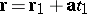and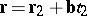has the form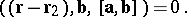The distance between two skew lines is the length of the segment of their common perpendicular whose end points lie on the lines (or, the distance between the two parallel planes containing the two lines). The distance between two skew lines is given by